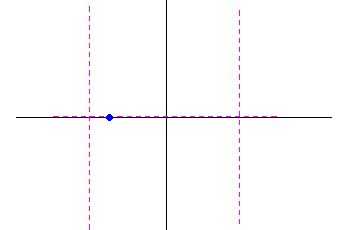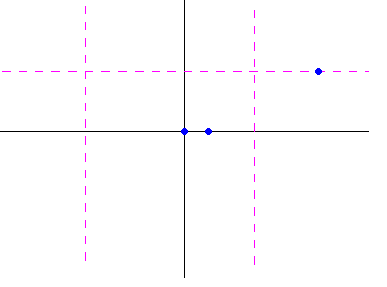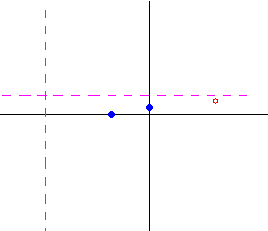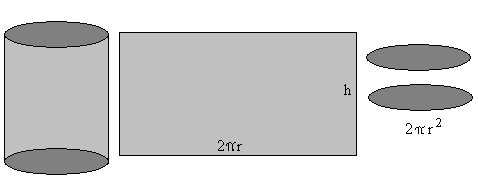The Graph of a Rational Function
List of things to do to analyze the graph of a rational function f (x) =
Find the domain
there are places where denominator = 0 are prohibited, otherwise domain is R.
Locate any intercepts
solve p(x) = 0. The x intercepts are points of the form (r,0) where
r is a root of p(x) but not a root of q(x).
Vertical asymptotes
reduce f(x) to lowest terms. Vertical asymptotes are lines of the form x = r
where r is a root of q(x) = 0.
Horizontal and oblique asymptotes
If the degree of q(x) is greater, then y = 0 is a horizontal asymptote.
If the degree of q(x) equals the degree of p(x) then y =
horizontal asymptote where p and q are the leading coefficients of p(x) and q(x). You can solve an equation to if the graph crosses the horizontal asymptote.
If the degree of p(x) exceeds the degree of q(x) by 1, then the from dividing p(x) by q(x) is an oblique asymptote. Test for symmetry
− ) = f (x) then f is symmetric about the y axis.
− (x) then f is symmetric about the origin. ex 1 After analysis, sketch the graph, then confirm with a grapher Domain R\{-4,4}
vertical asymptotes x = 4 and x = -4horizontal asymptote y = 0Try a values x = -5,-3.5,-2,4 to get the sign of the graph in each 'zone'. That should be enough. ex 2 Analyze the rational function, then sketch the graph and confirm with a grapher: Domain is R\{-4,3}
x intercepts are (0,0) and (1,0), y intercept is (0,0).
vertical asymptotes are x = -4 and x = 3, horizontal asymptote is y = 3Does the graph cross its asymptote? solve 3 =
3 x + x − 12 = 3x − 3xex 3 Construct a rational function f(x) that has x intercepts (3,0) and (5,0), vertical asymptotes x = 2 and x = -1, and a horizontal asymptote y = 5.
ex 4 Analyze and then sketch the graph of the rational function: The domain is R\{-5,3}
This one has a bit of a trap in it. There is no intercept at x = 3 nor is x=3 a vertical asymptote, but instead there is a hole in the graph at the point 3 ,  The x intercept is (-2,0), the y intercept is 0 ,  A vertical asymptote exists at x = -5 and a horizontal asymptote is y = 1 and since there are no solutions for 1 =ex 5 Reynolds aluminum makes cans in the shape of cylinders with capacity 500 mL (500 cubic centimeters). The top and bottom of each can are made from a special alloy costing .05 cents per square cm and the sides are made from material cosing .02 cents per centimeter. Express the cost of the material for a can as a function of the radius r of the can. Sketch the graph of C=C(r)What value of r leads to the least cost?What is that cost? Cost = 2πr (.05) + 2πr⋅h⋅(.02)
500 = π r h
C(r) = 2⋅π⋅r ⋅(.05) + 2⋅π⋅ 500
C(r) = 2⋅π⋅r ⋅(.05) + 2⋅
C(r) = .10⋅πr + r